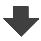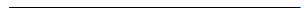Search:

# Reaction between nitric acid and copper

## Explanations

Use oxidation numbers to find the oxidant and the reductant!

$NO_3^-$ $(o.n.(N)=5)$ is the oxidant which is reduced to $NO$ $(o.n.(N)=2)$. $Cu$ $(o.n.(Cu)=0)$ is the reductant which is oxidised to $Cu^{2+}$ $(o.n.(Cu)=2)$.

How many electrons are exchanged by the oxidant and the reductant?

$NO_3^-$ catches 3 $e^-$. $Cu$ looses 2 électrons

Write the partial oxidation and reduction reactions in an acid solution.

$NO_3^-$ $+$ $3e^-$ $+$ $4H^+$ $\longrightarrow$ $NO$ $+$ $2H_2O$ $Cu$ $-$ $2e^-$ $\longrightarrow$ $Cu^{2+}$

Balance by the number of exchanged electrons and write the redox reaction!

$2NO_3^-$ $+$ $6e^-$ $+$ $8H^+$ $\longrightarrow$ $2NO$ $+$ $4H_2O$ $3Cu$ $-$ $6e^-$ $\longrightarrow$ $3Cu^{2+}$$2NO_3^-$ $+$ $3Cu$ $+$ $8H^+$ $\longrightarrow$ $3Cu{2+}$ $+$ $2NO$ $+$ $4H_2O$

Complete for the ions which are not involved et annotate!

$2H^+$ $+$ $2NO_3^-$ $+$ $3Cu$ $+$ $6H^+$ $+$ $6NO_3^-$ $\longrightarrow$ $3Cu^{2+}$ $+$ $6NO_3^-$ $+$ $2NO$ $+$ $4H_2O$ Nitric acid and copper produce a solution of copper(II) nitrate, nitrogen monoxide (colourless) and water.

Recall the reaction of nitrogen monoxide with the oxigen present in air! What is this type of reaction?

$2NO$(colourless gas)$+$ $O_2$ $\longrightarrow$ $2NO_2$ (brown gas) It is a redox reaction, because the o.n. of N goes from 2 to 4 at the cost of the oxigen atoms of the molecule $O_2$